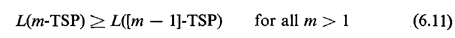### 6.4.11 m-TSP Problem

Keeping in mind that the m-traveling salesmen problem is, by definition, a single origin-destination problem, it can be easily shown that it can be reformulated as a classical TSP with little effort. Thus, the m-salesmen problem is no more difficult than its one-salesman counterpart, as measured by worstcase computational complexity. (Interestingly, this simple observation was not made until 1973 and 1974 in several independently published papers [BELL 74, ORLO 74, SVES 73].)

Suppose that a m-TSP is given with n points to be visited and with all tours beginning and ending at a common origin V. [Were m = I we would thus have a (n + I)-node TSP.] The equivalent formulation is then obtained by replacing the origin V by m exact copies of it, Vl, V2, . . . , Vm each connected to the other n nodes exactly as was the original origin and with the same distances. That is, if x is any one of the n points to be visited, then

d(V1, x) = d(V2, x) = . . . = d(Vm, x) = d(V, x)

However, the links connecting the m copies of the origin to each other are assigned "infinite" length, that is, very large by comparison to all other distances in the problem [d(Vi, Vj) =for all i, j = 1, 2, . . . , m].

If we now solve this as a classical single-salesman, (m + n)-point TSP, it can be seen that a minimum tour will never use a link connecting two copies of the origin. In other words, in the shortest single-salesman tour, the sequence "Vi followed by Vj" will never appear (i, j = 1, 2, . . . , m). Then, if the (m + n)-point, single-salesman tour is "folded back" by merging together all copies of the origin into a single node V, the single-salesman tour will decompose into m tours as required by the m-TSP.

Example 12: Two-Salesmen Problem

Consider the problem described by the (symmetric) distance matrix of Table 6-2. (The geometric problem on which the matrix is based is shown in Figure 6.29; ignore the indicated solution for the moment.)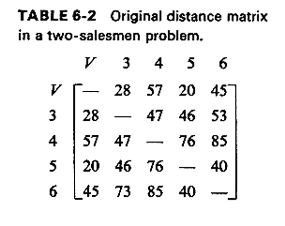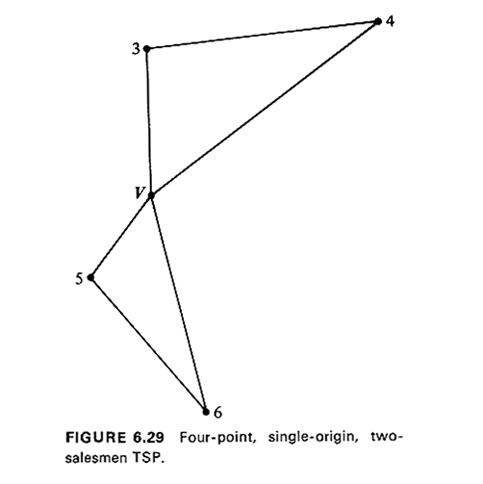Four points are to be visited from an origin V, using two salesmen. Hence, we need two copies of the origin V, which we call V1, and V2. The new distance matrix is then shown in Table 6-3. Note that the V1 and V2 rows (and columns) are identical and that we have set d(V1 V2) = d(V2, V1) =, i.e. to a "very large" number by comparison to other distances in a computer solution of the problem. (We have also set all distances from a point to itself to infinity, to prevent any "self-loops" no matter what solution method is used for the TSP.)

Table 6-3 now describes what is in effect a six-point single TSP. Careful consideration of the problem and some trial-and-error comparisons (or application of an exact TSP algorithm) Iead to the conclusion that the optimum solution of the TSP consists of the tour Vl-3-4-V2-5-6-V1, with a total length of 237 units. This tour is shown schematically in Figure 6.30. Merging V1 and V2 into the single original origin Vgives Figure 6.29, the solution to the two-TSP, with a length of 237 units.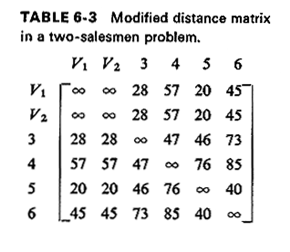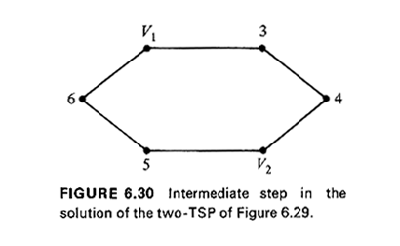With regard to the solution of the m-TSP problem, it should be noted that, theoretically, the "MST-and-matching" heuristic algorithm that we presented earlier (Algorithm 6.6) is not applicable, even when the original distance matrix is symmetric and satisfies the triangular inequality. The reason is that, because of setting the distances between the copies of the origins to infinity (or to a "very large" number), the expanded matrix--with the m - 1 copies of the origin--does not satisfy the triangular inequality.

In practice, however, if one is careful, the algorithm still can be applied with success (in the great majority of cases) to obtain a single TSP tour with the expanded matrix. The only cases when the algorithm fails occur when more than half of the odd nodes of the minimum spanning tree on completion of Step 1 of Algorithm 6.6 are nodes that correspond to copies of the origin. This, in turn, would mean that in matching odd degree nodes (Step 2) we would be forced to match two copies of the origin to each other--leading to a tour of infinite length.

We conclude our discussion of the m-TSP by noting that, irrespective of whether or not the original distance matrix satisfies the triangular inequality,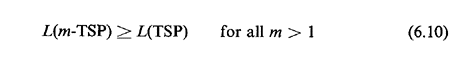where L(m-TSP) and L(TSP) are, respectively, the lengths of the optimum m-salesmen and single-salesman tours for any given problem. To see the validity of (6.10) simply note that the m-TSP tour has been shown here to cover m - 1 points in addition to the original n + 1 that the 1-TSP tour covers. In fact, we have shown by construction that, more generally,